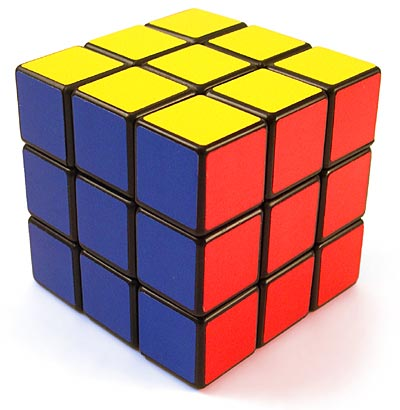A completely solved cube is one orientation of a Rubik’s Cube. Turning a face results in another … so how many total orientations of the Rubik’s Cube are there? To answer that, we need to look at how many possible combinations of corner and edge pieces are valid, in the sense that the cube is solvable. Later, I’ll discuss how many possible ways there are of combining the pieces randomly after disassembling the cube, which usually results in an unsolvable cube.There are six center “pieces” that do not move; they are fixed in place. Then we have the corners and edges that compose the rest of each of the six faces of the cube. There are eight corner pieces, which have three colors, and twelve edge pieces, which have two colors. Take a look at a Rubik’s cube and make sure you understand the distinction.

We think of having eight corner pieces to “fit” into eight corner “slots” in the Rubik’s cube, so there are 8! ways to position the corners. Each individual corner, though, has three faces, and they can be oriented in different ways while maintaining the same actual position on the cube. Therefore, there are 3^7 ways of orienting the faces of the corners. Notice that it’s not 3^8 because if we orient the faces of the first seven corners, then the eighth corner is fixed in place – it cannot be modified without changing the position of the faces on the other corners.

So far, that gives us 8!*3^7 possible orientations. But we now have to consider our 12 edges. We have 12 edges so there are 12! ways of positioning them, along with 2^11 orientations for their two faces. Again, we use n-1 edges where n=12 since the twelfth edge is forced in place if we don’t want to alter the first 11 edges. This implies that if we have a completely “solved” Rubik’s cube with the exception of one edge that is in the wrong orientation, we won’t ever be able to solve that cube since there’s no algorithm that can adjust the faces of one edge without messing up another part of the cube. (Well, you could take the cube apart if you want, but that is cheating.)

All together, we have 8!*3^7*12!*2^11 orientations of the cube. But this is actually double the amount we have, because we have to consider even vs. odd permutations. By this, we are talking about the amount of transpositions we have to perform. A transposition here refers to swapping two corners without altering the rest of the cube, or swapping two edges without altering the rest of the cube. A key concept is that the overall “sign” for the group of edges and the group of corners must be the same; both can be even, both can be odd, but they cannot differ. A group, by the way, is a set with an operation that satisfies properties of associativity, identity and invertibility, but that is beyond the scope of this article because no abstract algebra knowledge is assumed.

What does this mean? Suppose we have a cube that is almost solved. Also suppose that if we can perform 3 transpositions (or swaps) of the corner pieces, we will have correctly oriented all the remaining corners. This means that, if the cube is solvable, the number of swaps needed for the edge pieces must be odd, so the overall parity is even. We might also need 3 swaps of the edges to solve the cube, or we could use just 1 swap if necessary. It is impossible to solve a cube that has an overall parity that is odd.

Therefore, we have to divide what we computed before by half. So the number of possible orientations of a Rubik’s cube, under the assumption that the cube is solvable, is (8!*3^7*12!*2^11)/2 = 43,252,003,274,489,856,000. If we wanted to see the total number of orientations, regardless of whether the cube is solvable, we multiply this by 12. Only a third of the corner orientations are solvable, and only half of the edge orientations are solvable. And, of course, we have the even vs. odd permutations as I explained above. All together, 2*2*3 = 12.

The astute observer will realize that this means that if he has all the pieces of the Rubik’s cube scattered in front of him and assembles them randomly, he will have a 1 in 12 chance of creating a solvable Rubik’s Cube.